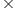Maths-
General
Easy

Question

# A teak wood log first cut in the form of a cuboid of length 2.3 m, width 0.75 m and of certain thickness. Its volume is of 1.004m^3. How many rectangular planks of size 2.3m x 0.75m x 0.04m can be cut from the cuboid

## 13141516Hint:

## The correct answer is: 14

### We are given the dimensions of teakwood log asLength l = 2.3 mBreadth b = 0.75Its volume = 1.004 m^3We have to find thickness h.And planks dimensions areLength = 2.3Breadth = 0.75Thickness = 0.04Volume of wood log = lbh1.004  = 2.30.75h1.004 = 1.725hh = 1.004/ 1.725h = 0.5820As the length and breadth are the same for both log and plank then we should divide the height by thickness of the plank so we will get number of planks.No of planks = 0.5820/ 0.04= 14.55~ 14Therefore the correct answer is b) 14.#### With Turito Foundation.#### Get an Expert Advice From Turito.